Industry Article

# Resolving the Signal Part 1: Introduction to Noise in Delta-Sigma ADCs

January 23, 2018 by Bryan Lizon, Texas Instruments

## This 12-part “Resolving the Signal” series aims to provide a comprehensive understanding of noise in delta-sigma ADCs. In part 1 of this series, I’ll focus on ADC noise fundamentals.

One of the fundamental challenges in any signal-chain design is ensuring that the system noise floor is low enough for the analog-to-digital converter (ADC) to resolve signals of interest. Regardless of your efforts to minimize power consumption, decrease board space or reduce cost, noise levels greater than the input signals render any design effectively useless. As a result, it’s fundamental knowledge for any analog designer to understand signal-chain noise, its effect on analog-to-digital conversion and how to minimize its impact.

To that end, this “Resolving the Signal” series aims to provide a comprehensive understanding of noise in delta-sigma ADCs. In these articles, I will examine common noise sources in a typical signal chain and complement this understanding with methods to mitigate noise and maintain high-precision measurements.

Before continuing on, it’s important to note here that this article series covers precision (noise), not accuracy. While the two terms are often used interchangeably, they refer to different – though related – aspects of signal-chain design. When designing high-performance data-acquisition systems, you must also consider errors due to inaccuracy, such as offset, gain error, integral nonlinearity (INL) and drift, in addition to minimizing noise.

In part 1 of this series, I’ll focus on ADC noise fundamentals while answering questions and discussing topics such as:

• What is noise?
• Where does noise come from in a typical signal chain?
• Understanding inherent noise in ADCs.
• How is noise different in high-resolution vs. low-resolution ADCs?

In part 2, I’ll shift the focus to these topics:

• Noise specifications in ADC data sheets.
• Absolute vs. relative noise parameters.

In part 3, I’ll step through a complete design example using a resistive bridge to help illustrate how the theories from parts 1 and 2 apply to a real-world application.

### What is Noise and Where Does it Come From?

Noise is any undesired signal (typically random) that adds to the desired signal, causing it to deviate from its original value. Noise is inherent in all electrical systems, so there is no such thing as a “noise-free” circuit.

Figure 1 depicts how you might experience noise in the real world: an image with the noise filtered out and that same image with no filtering. Note the crisp detail in the left image of Figure 1, while the right image is almost completely obscured. In the analog-to-digital conversion process, the result would be information loss between the analog input and the digital output, much like how the two images in Figure 1 bear virtually no resemblance to each other.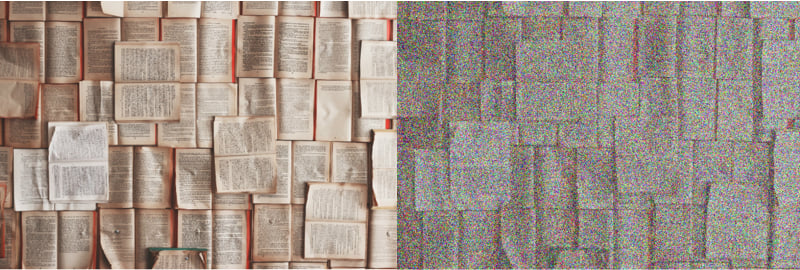##### Figure 1: A noise-free image (left); the same images with noise (right)

In electronic circuits, noise comes in many forms, including:

• Broadband (thermal, Johnson) noise, which is temperature-dependent noise caused by the physical movement of charge inside electrical conductors.
• 1/f (pink, flicker) noise, which is low-frequency noise that has a power density inversely proportional to frequency.
• Popcorn (burst) noise, which is low frequency in nature and caused by device defects, making it random and mathematically unpredictable.

These forms of noise may enter the signal chain through multiple sources including:

• ADCs, which contribute a combination of thermal noise and quantization noise.
• Internal or external amplifiers, which can add broadband and 1/f noise that the ADC then samples, allowing it to affect the output code result.
• Internal or external voltage references, which also contribute broadband and 1/f noise that appears in the ADC’s output code.
• Non-ideal power supplies, which may add noise into the signal you’re trying to measure with several means of coupling.
• Internal or external clocks, which contribute jitter that translates into non-uniform sampling. This appears as an additional noise source for sinusoidal input signals and is generally more critical for higher-speed ADCs.
• Poor printed circuit board (PCB) layouts, which can couple noise from other parts of the system or environment into sensitive analog circuitry.
• Sensors, which can be one of the noisiest components in high-resolution systems.

Figure 2 depicts these noise sources in a typical signal chain.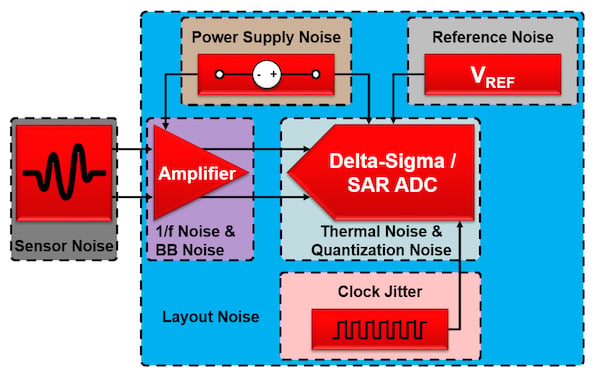##### Figure 2. Common noise sources in a typical supply chain

In parts 1-3 of this 9-part series, I’ll focus on inherent ADC noise only. For a more comprehensive understanding, I’ll discuss the sources of noise in the remaining circuit components in separate articles.

You can break total ADC noise into two main sources: quantization noise and thermal noise. These two noise sources are uncorrelated, which enables the root-sum-squares (RSS) method to determine the total ADC noise, NADC, Total, as shown in Equation 1: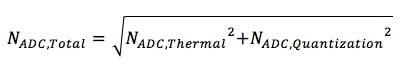Each ADC noise source has particular properties that are important when understanding how to mitigate inherent ADC noise.

#### Quantization Noise

Figure 3 depicts the plot of an ADC’s ideal transfer function (unaffected by offset or gain error). The transfer function extends from the minimum input voltage to the maximum input voltage horizontally and is divided into a number of steps based off the total number of ADC codes along the vertical axis. This particular plot has 16 codes, or steps, representing a 4-bit ADC. (Note: An ADC using straight binary code would have a transfer function that only included the first quadrant.)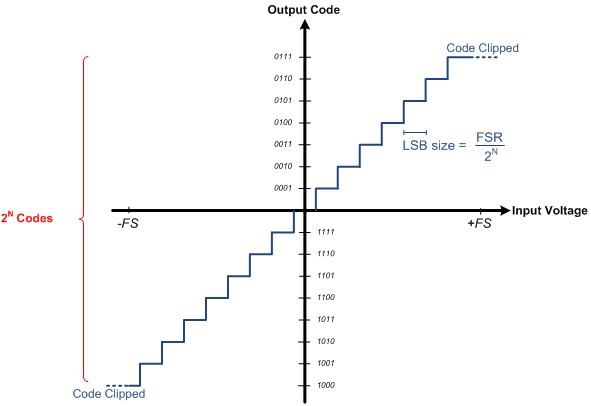##### Figure 3. An ADC's ideal transfer function

Quantization noise comes from the process of mapping an infinite number of analog voltages to a finite number of digital codes. As a result, any single digital output can correspond to several analog input voltages that may differ by as much as ½ least significant bit (LSB), which is defined in Equation 2: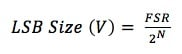where FSR represents the value of the full-scale range in volts and N is the ADC’s resolution.

If you map this LSB error relative to a quantized AC signal, you’ll get a plot like the one shown in Figure 4. Note the dissimilarity between the quantized, “stair-step”-shaped digital output and the smooth, sinusoidal analog input. Taking the difference between these two waveforms and plotting the result yields the “sawtooth”-shaped error shown at the bottom of Figure 4. This error varies between ±½ LSB and appears as noise in the result.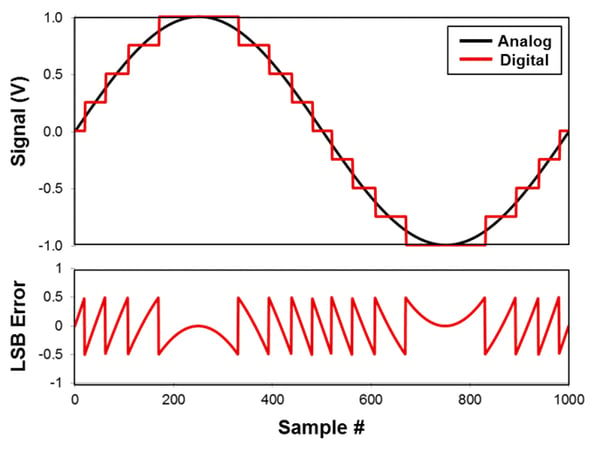Similarly, for DC signals, the error associated with quantization varies between ±½ LSB of the input signal. However, since DC signals have no frequency component, quantization “noise” actually appears as an offset error in the ADC output.

Finally, an obvious but important result of quantization noise is that the ADC cannot measure beyond its resolution, as it cannot distinguish between sub-LSB changes in the input.

#### Thermal noise

Unlike quantization noise, which is a byproduct of the analog-to-digital (or digital-to-analog) conversion process, thermal noise is a phenomenon inherent in all electrical components as a result of the physical movement of charge inside electrical conductors. Therefore, you can measure thermal noise even without applying an input signal.

Unfortunately, ADC end users cannot affect the device’s thermal noise because it is a function of the ADC’s design. Throughout the rest of this article, I’ll refer to all ADC noise sources other than quantization noise as the ADC’s thermal noise.

Figure 5 depicts thermal noise in the time domain, which typically has a Gaussian distribution.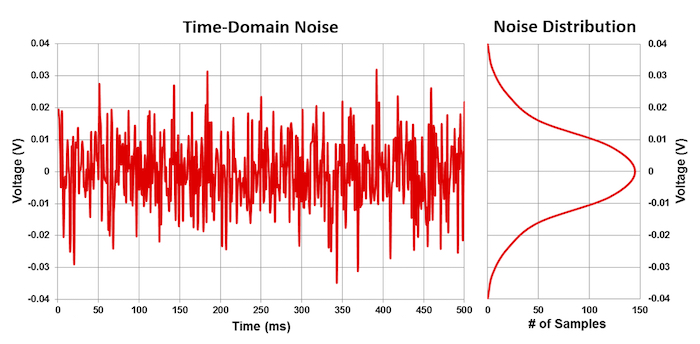##### Figure 5. Thermal noise in the time domain with Gaussian distribution

Although you cannot affect the ADC’s inherent thermal noise, you can potentially change the ADC’s level of quantization noise due to its dependence on LSB size. However, quantifying the significance of this change depends on whether you’re using a “high-resolution” or a “low-resolution” ADC. Let’s quickly define these two terms so that you can better understand how to use LSB size and quantization noise to your advantage.

A low-resolution ADC is any device whose total noise is more dependent on quantization noise such that NADC,Quantization >> NADC,Thermal. Conversely, a high-resolution ADC is any device whose total noise is more dependent on thermal noise, such that NADC,Quantization << NADC,Thermal. The transition between low and high resolution typically occurs at the 16-bit level, with anything >16 bits considered high resolution and anything <16 bits considered low resolution. While not always true, I’ll keep this general convention throughout the remainder of this series.

Why make this distinction at the 16-bit level? Let’s look at two ADC data sheets to find out. Figure 6 shows the actual noise tables for Texas Instruments’ ADS114S08, a 16-bit delta-sigma ADC, and its 24-bit counterpart, the ADS124S08. Other than their resolutions, these ADCs are identical.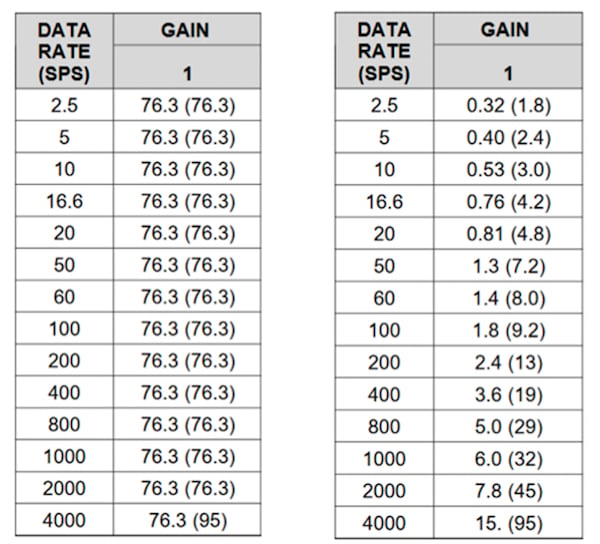##### Figure 6. Input-referred noise for the 16-bit ADS114S08 (left) and 24-bit ADS124S08 (right) in µVRMS (µVPP) at VREF = 2.5V, G = 1V/V

In the noise table for the 16-bit ADS114S08, all of the input-referred noise voltages are the same regardless of data rate. Compare that to the 24-bit ADS124S08’s input-referred noise values, which are all different and decrease/improve with decreasing data rates.

While this doesn’t result in any definitive conclusions by itself, let’s use Equations 3 and 4 to calculate the LSB size for each ADC, assuming a 2.5V reference voltage: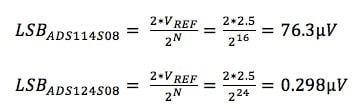Combining these observations, you can see that the low-resolution (16-bit) ADC’s noise performance as reported in its data sheet is equivalent to its LSB size (maximum quantization noise). On the other hand, the noise reported in the high-resolution (24-bit) ADC’s data sheet is clearly much larger than its LSB size (quantization noise). In this case, the high-resolution ADC’s quantization noise is so low that it’s effectively hidden by the thermal noise. Figure 7 below represents this comparison qualitatively.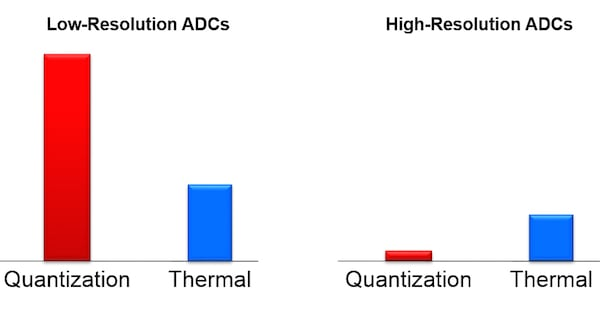##### Figure 7. Qualitative representation of quantization noise and thermal noise in low-resolution (left) and high-resolution (right) ADCs

How can you use this result to your advantage? For low-resolution ADCs where quantization noise dominates, use a smaller reference voltage to reduce the LSB size, which reduces the quantization noise amplitude. This has the effect of lowering the ADC’s total noise, represented by Figure 8 (left).

For high-resolution ADCs where thermal noise dominates, use a larger reference voltage to increase the input range (dynamic range) of the ADC, while ensuring that the quantization noise level remains below the thermal noise. Assuming no other system changes, this increased reference voltage enables a better signal-to-noise ratio, which you can see in Figure 8 (right).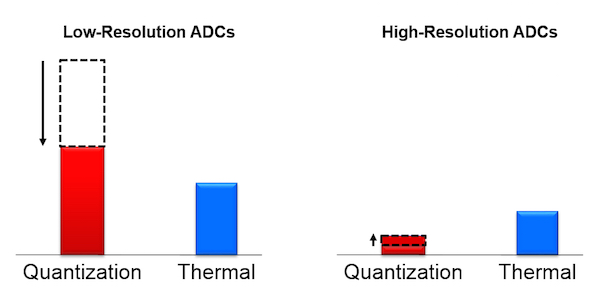##### Figure 8. Adjusting quantization noise in low-resolution (left) and high-resolution (right) ADCs to improve performance

Now that you understand the components of ADC noise and how they vary between high- and low-resolution ADCs, you can use this knowledge for part 2, where I’ll discuss how noise is measured and specified in an ADC’s data sheet.

### Key Takeaways

Here is a summary of important points to help better understand noise in delta-sigma ADCs:

• Noise is inherent in all electrical systems.
• Noise is introduced via all signal-chain components.
• There are two main types of ADC noise:
• Quantization noise, which scales with reference voltage.
• Thermal noise, which is a fixed value for a given ADC.
• One type of noise generally dominants depending on the ADC’s resolution:
• Thermal noise-dominated.
• The resolution is typically >1 LSB.
• Increase the reference voltage to increase the dynamic range.•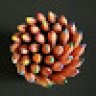Cemra JC December 20, 2018